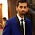Programming Geek
Rated 4.7/5 based on 1446 reviews### CodeVita 2013 : Sequence (Longest Progressive Sequence)

Given a sequence, the objective is to find the longest progressive sequence arranged in ascending order. Detailed descriptions are as:

Problem
A sequence is said to be progressive if it doesn’t decrease at any point in time.
For example 1 1 2 2 is a progressive sequence but 1 2 1 is not a progressive sequence. Let S be the sequence and be represented by L spaced integers Ki, now your task is to find out the first longest progressive sequence present in the given sequence (S).

Input Format:

First line will contain T, the length of the sequence and next line will contain T spaced integers Ki (where i = 0,1, …,L).
Line 1 T,where T is the length of the sequence
Line 2 Ki,where Ki is integer in sequence separated by space

Constraints:

1<=T<=10^6(one million)
1<=Ki<=10^9(one billion)

Output Format:

Line 1 longest progressive sequence present in the given sequence

Sample Test Cases:

SNo.InputOutput
1
4
1 1 2 1
1 1 2
2
5
1 2 1 2 2
1 2 2

I have not followed coding standards while solving the problems.

```/**
*
* @author VIK VIKASH VIKKU
*/
import java.util.ArrayList;
import java.util.Scanner;

public class LongestProgressiveSequence {

public static void main(String[] args) {
Scanner sc = new Scanner(System.in);
int t = sc.nextInt();
ArrayList<Integer> ls = new ArrayList<Integer>();
Integer[] temp = new Integer;
for (int i = 0; i < t; i++) {
int num = sc.nextInt();
if (!(ls.isEmpty())) {
if (num >=ls.get(ls.size()-1 )) {

} else {

if(ls.size()>temp.length)
temp=ls.toArray(temp);
ls.clear();
}

} else {
}
}

if(ls.size()>temp.length)
{
temp=ls.toArray(temp);
ls.clear();
}
for (int i = 0; i < temp.length; i++) {
if(i<temp.length-1)
System.out.print(temp[i]+" ");
else
System.out.println(temp[i]);

}

}
}

```

#### 1 comment :

1.can i get a solution in C++ .....!!!!!!